# Anaerobic & Aerobic Treatment Biochemical Kinetics Notes | Study Environmental Engineering - Civil Engineering (CE)

## Civil Engineering (CE): Anaerobic & Aerobic Treatment Biochemical Kinetics Notes | Study Environmental Engineering - Civil Engineering (CE)

The document Anaerobic & Aerobic Treatment Biochemical Kinetics Notes | Study Environmental Engineering - Civil Engineering (CE) is a part of the Civil Engineering (CE) Course Environmental Engineering.
All you need of Civil Engineering (CE) at this link: Civil Engineering (CE)

BASIC EQUATION FOR BIOLOGICAL TREATMENT OF WASTEWATER

According to Monod kinetics,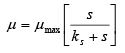(4.2.1)
Where, s is the substrate concentration, ks is the substrate concentration when µ(=µmax/2), µmax is the maximum µ when substrate is not limiting. Also, solid production rate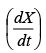is related to substrate utilization rate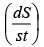by
following relationship: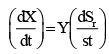(4.2.2)
Where, Sr is the mass of soluble substrate (i.e. BOD), Y is the yield coefficient (kg of new cells formed/kg BOD removed). However owing to large treatment time in many of the large treatment units, substantial number of cells may die because of endogenous respiration. Therefore,

Net production rate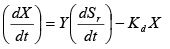(4.2.3)

Where, Kd is the endogenous respiration decay rate constant. For growth phase only,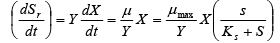(4.2.3)

Case 1- S>>Ks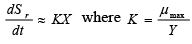(4.2.4)
i.e. removal rate is independent of substrate concentration and that the removal rate depends on X only.

Case 2: S<< KS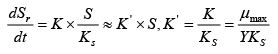(4.2.5)

Here, removal rate depends both upon X and S. Where, X is the mass of biomass in the system (usually represented by MLSS i.e. Mixed Liquor Suspended Solid), µ is the specific growth rate constant (time-1).

Major Terms [a] Hydraulic detention time,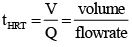(4.2.6)

[b] Sludge age or mean residence time(θC)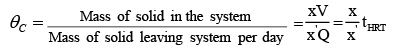(4.2.7)

Where, x (=X/V) the concentration of microbial solution in the system, x’ is the concentration of solids withdrawn.

For the flow through system, x’ = x and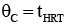For the flow system with recycling, x’ < x and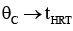[c] Food to microorganism ratio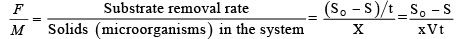(4.2.8)

Problem 4.2.1: An aerated activated sludge tank is being operated under following conditions: Q=4400 m3/d, MLSS=3500 mg/l, Y=0.5, tank volume =770 m3, Endogeneous decay rate constant kd=0.09 d-1.
(a) Estimate weight of solids produced per day for the conditions in which BOD is reduced from 350 mg/l to 130 mg/l.
(b) Estimate θc.
(c) Estimate F/M ratio.

Solution: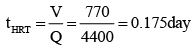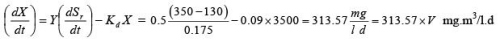=241.4 kg/d.

θc  = mass of solid in the system/ mass of solid leaving the system per day =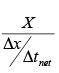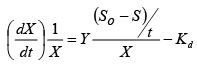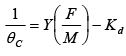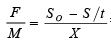= 0.359 (kg BOD/kg MLSS)

θc = 11.17 day.

The document Anaerobic & Aerobic Treatment Biochemical Kinetics Notes | Study Environmental Engineering - Civil Engineering (CE) is a part of the Civil Engineering (CE) Course Environmental Engineering.
All you need of Civil Engineering (CE) at this link: Civil Engineering (CE)Use Code STAYHOME200 and get INR 200 additional OFF

## Environmental Engineering

14 videos|74 docs|70 tests

Track your progress, build streaks, highlight & save important lessons and more!

,

,

,

,

,

,

,

,

,

,

,

,

,

,

,

,

,

,

,

,

,

;